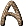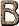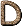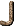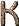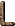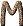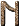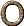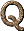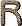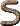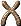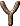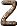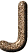J-file A group of lines of a supermultiplet having a common lower level. [H76] j-j Coupling see LS coupling [H76] J magnitude The magnitude derived from the observations at an infrared wavelength of 1.3 microns. [H76] J Meson name for the J- or-meson, mass 3 GeV, composed of a charmed quark and charmed antiquark. Its discovery in 1974 instigated a scientific revolution in the later half of the decade. [D89] J-value Value of the total angular momentum (orbital plus spin). J is the rotational quantum number which specifies the rotational level of a molecule. [H76] Jacobi Ellipsoid Jacobi discovered that homogeneous, self-gravitating masses rotating uniformly and sufficiently rapidly can have the shape of triaxial ellipsoids. These are the Jacobi ellipsoids. [H76] Jansky A unit used in radio astronomy to indicate the flux density of electromagnetic radiation received from outer space, one jansky being equal to 10-26 W m-2 Hz-1. The unit is named after the American electrical engineer Karl G. Jansky (1905-1950), who became the first radio astronomer in the world in December 1930 when he detected electromagnetic radiation of wavelength 15 m coming from the Milky Way (see Flux unit). It was adopted in August 1973 by the International Astronomical Union. [JM92] (b) Unit of flux density adopted by the IAU in 1973. 1 Jy = 10-26 W m-2 Hz-1. [H76] Janus The innermost satellite of Saturn, just outside Saturn's rings. P = 0.75 days; R = 175 (?) km; i0; e0. It was discovered by Dollfus in 1966 and was named Janus for the first and the last. [H76] JD Julian Day -- A unit of time within the Julian Dating System where the number of ephemeris days that have elapsed since 12h ephemeris time on January 1, 4713 B.C. JD for 1970 January 1 is 2440588. Jeans Instability Criterion (below) see Jeans Length Jeans Length (a) The critical wavelength (J = cs (/ G0)1/2, where cs is the isothermal sound speed in the medium) at which the oscillations in an infinite, homogeneous medium become gravitationally unstable. Any disturbance greater than the Jeans length will decouple by self-gravitation from the rest of the medium to become a stable, bound system. In general,J1020 cm. [H76] Jeans Mass (a) The critical mass a volume of space must contain before it will collapse under the force of its own gravity. [C97] (b) The mass enclosed within a sphere of diameter equal to the Jeans length. [Silk90] Jet Spray of particles produced from the vacuum by the passage of a high momentum quark or gluon. The direction of the jet indicates the direction of the said quark or gluon. [D89] J H K L M N Q Designations for parts of the infrared waveband transmitted by the Earth's atmosphere in the wavelength range 1 to 20 µm. [McL97] JPL Jet Propulsion Laboratory, Pasadena, California. Funded by NASA and operated by the California Institute of Technology (Caltech). [McL97] Jitter Irregular random variations in a radio signal. [H76] Jodrell Bank A telescope in England designed to detect radio waves emitted by astronomical objects. [LB90] Johnson Noise Low-frequency electromagnetic radiation associated with thermal fluctuations, which is emitted by all bodies whose temperature is above 0 K. [H76] Josephson Effect Any of various electrical effects observed with pairs of superconductors. Josephson junctions (between semiconductors) are finding various applications in investigating fundamental constants, the phenomenon of electron tunneling, and in high-speed computers. [DC99] Joule (a) The SI unit of energy, work, or quantity of heat. 1 J is equal to a force of 1 newton acting over a distance of 1 meter. 1 J = 107 ergs. [H76] (b) The SI and the practical unit of work which is equal to a force of one newton acting over a distance of one metre in the direction of the force. It is equivalent to 107 ergs and in electrical units is equal to a watt second. The unit was originally proposed by the British Association in 1888, who recommended that it be named after J. P. Joule (1818-1889), the originator of the mechanical theory of heat. It was recognized by the IEC in 1889. In 1948 the joule was adopted as the unit of heat by the International Conference on Weights and Measures, so that the specific heat of water at 15°C is 4185.5 joule (kg °C)-1, a figure previously always associated with J, the mechanical equivalent of heat. The idea of using the joule as a unit of heat had the support of the British Association in 1896, but little notice was taken of their proposal at that time. (1 J = 2.78 × 10-7 kW h = 9.47 × 10-4 Btu = 9.47 × 10-9 therm.) [JM92] Joule Dissipation The heat produced when a current is passed through an electrically resisting medium. [H76] Jovian Giant planets that have a gaseous surface; the sun's known Jovian planets are Jupiter, Saturn, Uranus, and Neptune. [F88] Julian Calendar (a) Calendar established by Julius Caesar in 46 BC, which overestimated the duration of the sidereal year by 11 minutes and 14 seconds. It was replaced, from 1582, by the Gregorian calendar, by which time it was inaccurate by a total of 10 days. [A84] (b) The calendar introduced by Julius Caesar in 46 B.C. to replace the Roman calendar. In the Julian calendar a common year is defined to comprise 365 days, and every fourth year is a leap year comprising 366 days. The Julian calendar was superseded by the Gregorian calendar. [S92] Julian Date (a) JD: The number of ephemeris days that have elapsed since 12h ephemeris time on January 1, 4713 B.C. JD for 1970 January 1 is 2440588. [H76] (b) The interval of time in days and fraction of a day since 4713 B.C. January 1, Greenwich noon, Julian proleptic calendar. In precise work the timescale, e.g., dynamical time or Universal Time, should be specified. [S92] Julian Date, Modified MJD -- The Julian Date minus 2400000.5. [S92] Julian Proleptic Calendar The calendric system employing the rules of the Julian calendar, but extended and applied to dates preceding the introduction of the Julian calendar itself. [S92] Julian Year A period of 365.25 days. This period served as the basis for the Julian calendar. [S92] Jump Conditions The conditions for jumps in pressure and density (or temperature or energy) across a shock wave. These are the Hugoniot conditions. [H76] Juno An asteroid 250 km in diameter (P = 1,594 days; a = 2.67 AU: e = 0.256; i = 13°.0) with a relatively large albedo (0.2). Rotation period 7h.21. [H76] Jupiter Fifth planet from the Sun. Mass 1.90 × 1030 g = 318 times Earth's. It is more massive than all other planets and satellites combined; if it were about 80 times more massive, it would become self-luminous. Equatorial radius 7.135 × 109 cm = 11 times Earth's; polar radius 6.7 × 109 cm; oblateness (from Pioneer 10) 0.065. Surface gravity 2.7 that of Earth; Vesc 61 km s-1; mean density 1.33 g cm-3. Rotational period 9h50m at equator; 9h55m at polar regions (see Systems I and II longitude). Semi-major axis 5.203AU, e = 0.048, i = 1°18'18". Obliquity 3°.1. Orbital period 11.86 years, mean orbital velocity 13.06 km s-1. Synodic period 398.9 days. Albedo 0.51. Surface temperature about 120 K. Current estimates of Jupiter's central temperature and density are 5 × 104 K and about 35 megabars (the heat flux to the surface is mainly convective). For both Jupiter and Saturn it is necessary to invoke a substantial source of internal heating (presumably gravitational contraction) to account for the surface temperature (Jupiter radiates about 2 1/2 times as much heat as it receives from the Sun). Jupiter's core is probably metallic hydrogen in a pressure-ionized liquid phase. Jupiter's surface shows pronounced horizontal striations: the light layers (zones) are at a slightly higher altitude and about 15° cooler than the dark layers (belts). Atmosphere primarily H2 (85%) and He (14%), with traces of methane, ammonia, etc. Pioneer 10 established that Jupiter has a magnetic field of about 4 gauss (the magnetic axis is inclined 15° to the rotational axis and is offset about 0.1 Jupiter radius from the center of the planet) and also that it is surrounded by a partial torus of atomic H in the orbit of Io. Thirteen satellites, the four outermost of which have retrograde motion, high eccentricity, and high inclination. (Jupiter XIII, discovered in 1974, has a period of 239 days; i = 26°.7, e = 0.147.)